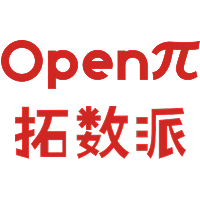# 数据科学在文本分析中的应用 ：中英文 NLP（上）2023-03-14

《后疫情时代，数据科学赋能旅游行业服务质量提升》这篇博文中，我们介绍了猫途鹰文本分析项目的背景和解决方案，并展示了最终的分析结果。接下来，对于中英文 NLP 感兴趣的读者，我们会为大家详细讲解数据采集、数据入库、数据清理和数据建模步骤中涉及的原理和代码实现。由于篇幅的限制，上篇会重点讲解数据采集、数据入库和数据清理这三个步骤，下篇则会讲解数据建模的完整流程。

## 数据采集

### 1.抓取工具分析

Beautiful Soup

Beautiful Soup 是几种工具中最容易上手的网页抓取库，它可以快速帮助开发者从 HTML 或 XML 格式的文件中获取数据。在这个过程中，Beautiful Soup 会一定程度上读取这类文件的数据结构，并在此基础上提供许多与查找和获取数据内容相关的方程。除此之外，Beautiful Soup 完善、易于理解的文档和活跃的社区使得开发者不仅可以快速上手，也能快速精通，并灵活运用于开发者自己的应用当中。

Scrapy

Scrapy 是非常受欢迎的开源网页抓取库之一，它最突出的特性是抓取速度快，又因为它基于 Twisted 异步网络框架，用户发送的请求是以无阻塞机制发送给服务器的，比阻塞机制更灵活，也更节省资源。因此，Scrapy 拥有了以下这些特性：

• 对于 HTML 类型网页，使用XPath或者CSS表述获取数据的支持
• 可运行于多种环境，不仅仅局限于 Python。Linux、Windows、Mac 等系统都可以使用 Scrapy 库。
• 扩展性强
• 速度和效率较高
• 需要的内存、CPU 资源较少

Selenium

Selenium 的起源是为了测试网页应用程序而开发的，它获取网页内容的方式与其他库截然不同。Selenium 在结构设计上是通过自动化网页操作来获取网页返回的结果，和 Java 的兼容性很好，也可以轻松应对 AJAX 和 PJAX 请求。和 Beautiful Soup 相似，Selenium 的上手相对简单，但与其他库相比，它最大的优势是可以处理在网页抓取过程中出现的需要文本输入才能获取信息、或者是弹出页面等这种需要用户在浏览器中有介入动作的情况。这样的特性使得开发者对网页抓取的步骤更加灵活，Selenium 也因此成为了最流行的网页抓取库之一。

### 2.网页数据和结构的初步了解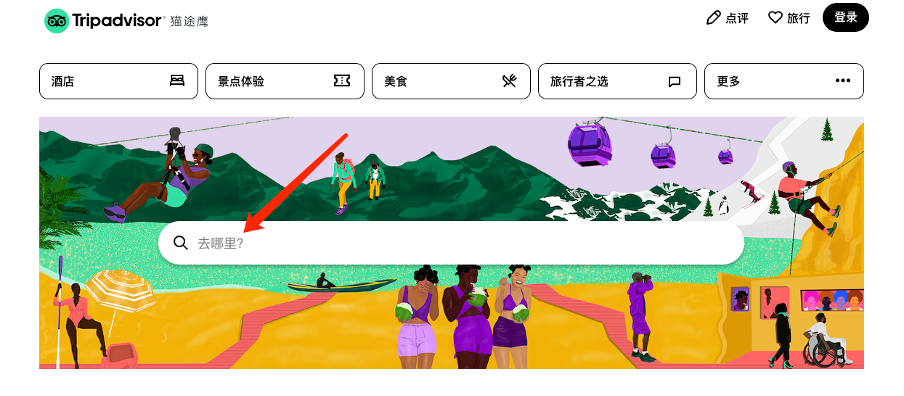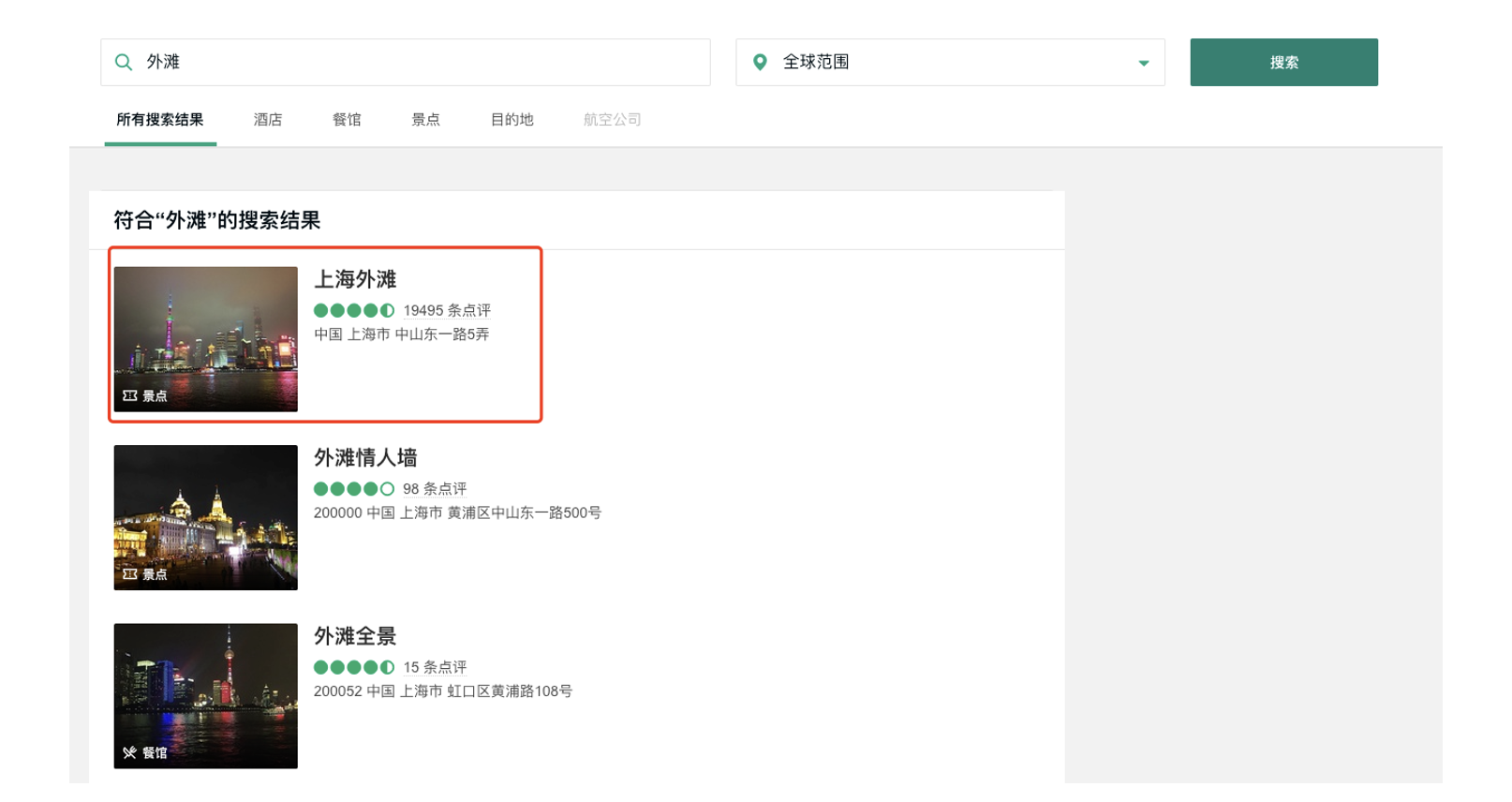• 猫途鹰的搜索引擎本身会对景点名称和搜索输入进行比较，通过自己内部的逻辑将符合条件的景点排名靠前
• 我们可以在结果出现后使用省份、城市等信息筛选得到目标景点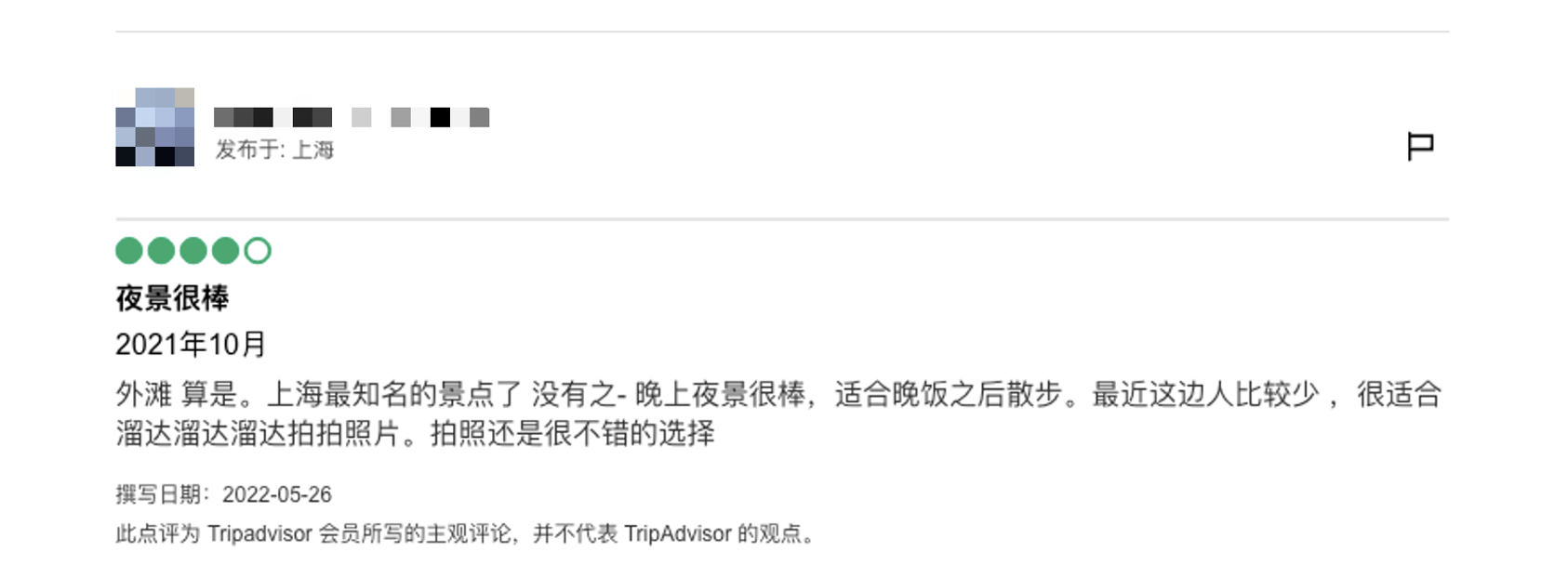• 用户
• 用户所在地
• 评分
• 点评标题
• 到访日期
• 旅行类型
• 详细点评
• 撰写日期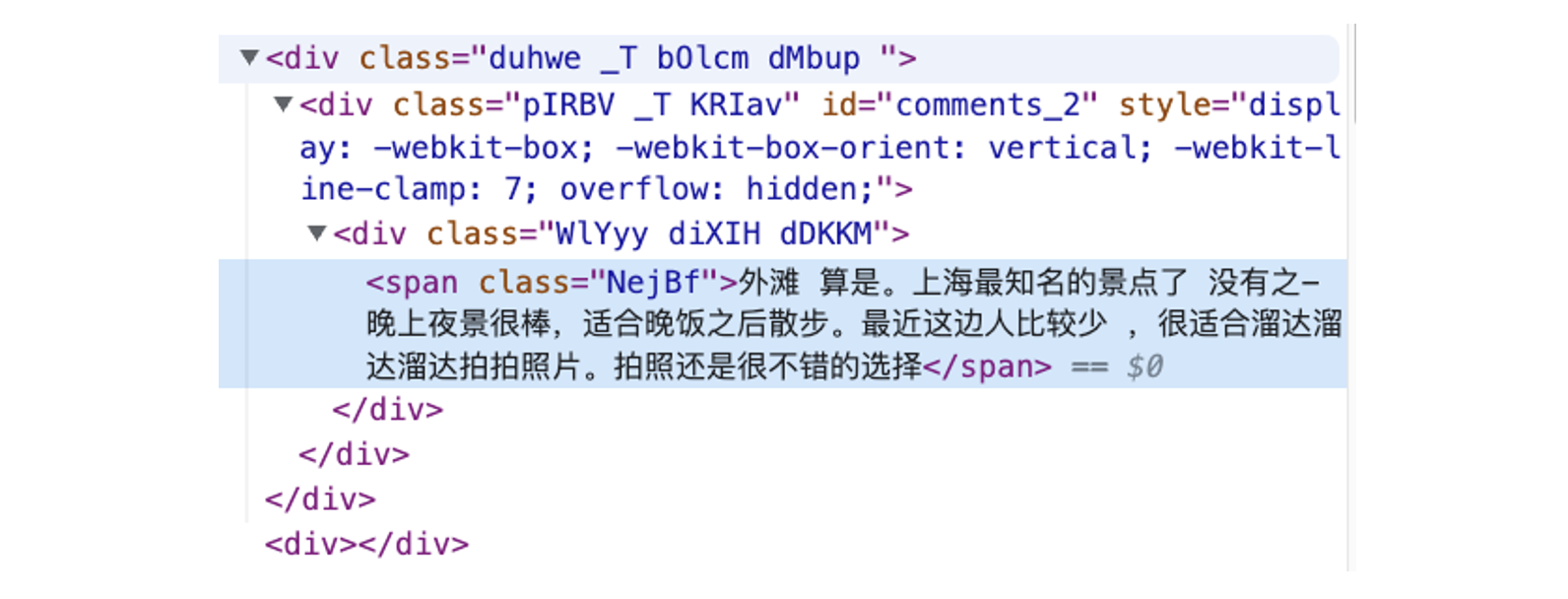• 第一步：发送请求，使用 Selenium 操作浏览器找到指定景点的评论页面
• 第二步：进入评论页面，抓取评论数据

### 3.获取评论数据

```from selenium import webdriver
import chromedriver_binary
from webdriver_manager.chrome import ChromeDriverManager
from selenium.webdriver.common.by import By
from selenium.webdriver.common.keys import Keys
import time
import datetime
import re
import pandas as pd
```

```#utility.py
import time
import configparser
def print_log_message(app_name, procedure, message):
ts = time.localtime()
print(time.strftime("%Y-%m-%d %H:%M:%S", ts) + " **" + app_name + "** " + procedure + ":", message)
return
config = configparser.ConfigParser()
var_value = config.get(section, var)
return var_value
```

```# Initiate web session
chrome_options = webdriver.ChromeOptions()
wd = webdriver.Chrome(ChromeDriverManager().install(),chrome_options=chrome_options)
wd.get(self.web_url)
wd.implicitly_wait(5)
review_results = {}
```

```location_name = '外滩'
city = '上海'
state = '上海'
```

```# Find search box
wd.find_element(By.CSS_SELECTOR, '.weiIG.Z0.Wh.fRhqZ>div>form>input').click()
```

```# Enter location name
wd.find_element(By.XPATH, '//input[@placeholder="去哪里？"]').send_keys(f'{location_name}')
wd.find_element(By.XPATH, '//input[@placeholder="去哪里？"]').send_keys(Keys.ENTER)
```

```# Find the right location with city + province info
element = wd.find_element(By.XPATH,
f'//*[@class="address-text" and contains(text(), "{city}") and contains(text(), "{state}")]')
element.click()
```

```comment_section = wd.find_element(By.XPATH, '//*[@data-automation="WebPresentation_PoiReviewsAndQAWeb"]')
```

```# user id
user_elements = comment_section.find_elements(By.XPATH, '//div[@class="ffbzW _c"]/div/div/div/span[@class="WlYyy cPsXC dTqpp"]')
user_list = [x.text for x in user_elements]
```

```# location
loca_elements = comment_section.find_elements(By.XPATH,
'//div[@class="ffbzW _c"]/div/div/div/div/div[@class="WlYyy diXIH bQCoY"]')
loca_list = [x.text[5:] for x in loca_elements]
```

```# trip type
trips_element = comment_section.find_elements(By.XPATH, '//*[@class="eRduX"]')
trip_types = [self.separate_trip_type(x.text) for x in trips_element]
```

```# comment date
```

```# drop out the first element
```

```# rating
rating_element = comment_section.find_elements(By.XPATH,
'//div[@class="dHjBB"]/div/span/div/div[@style="display: block;"]')
rating_list = []
for rating_code in rating_element:
code_string = rating_code.get_attribute('innerHTML')
s_ind = code_string.find(" bubble_")
rating_score = code_string[s_ind + len(" bubble_"):s_ind + len(" bubble_") + 1]
rating_list.append(rating_score)
```

```# comments title
'//*[@class="WlYyy cPsXC bLFSo cspKb dTqpp"]')
```

```# comments content
'//*[@data-automation="WebPresentation_PoiReviewsAndQAWeb"]'
).find_elements(By.XPATH, '//*[@class="duhwe _T bOlcm dMbup "]')
```

```# if review contains pictures
pic_sections = comment_section.find_elements(By.XPATH,
'//div[@class="ffbzW _c"]/div[@class="hotels-community-tab-common-Card__card--ihfZB hotels-community-tab-common-Card__section--4r93H comment-item"]')
pic_list = []
for r in pic_sections:
if 'background-image' in r.get_attribute('innerHTML'):
pic_list.append(1)
else:
pic_list.append(0)
```

```#引入之前定义的Python Class：
from data_processor import WebScrapper
scrapper = WebScrapper()
```

```#运行网页抓取方程抓取中文语料：
trip_review_data = scrapper.trip_advisor_zh_scrapper_runner(location, location_city, location_state, page_n=int(n_pages))
```

## 数据入库

```from sqlalchemy import create_engine
import psycopg2
```

```data.to_sql(table_name, engine, if_exists=‘replace’, index=False)
```

data 是我们需要入库的 Pandas DataFrame 数据，table_name 是表名，engine 是我们之前定义的 SQLAlchemy 引擎, if_exists=‘replace’ 和 index=False 则是 Pandas to_sql() 方程的选项。这里选项的含义是，如果表已存在则用现有数据替代已有数据，并且在入库过程中，我们不需要考虑索引。

## 数据清洗

• 用户信息（如所在地等）
• 评论信息（如是否包含图片信息等）
• 评论语料

```import numpy as np
import pandas as pd
import psycopg2
from sqlalchemy import create_engine
import langid
import re
import emoji
from sklearn.preprocessing import MultiLabelBinarizer
import demoji
import random
from random import sample
import itertools
from collections import Counter
import matplotlib.pyplot as plt
```

```df = pd.read_sql('SELECT * FROM "上海_上海_外滩_source_review"', engine)
df.shape
```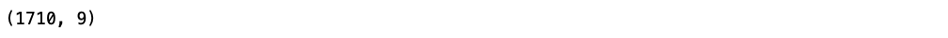```df = pd.read_sql('SELECT * FROM "Shanghai_Shanghai_The Bund (Wai Tan)_source_review_EN"', engine)
df.shape
```### 1.数据类型处理

```df['comment_date'] = pd.to_datetime(df['comment_date'])
df['rating'] = df['rating'].astype(str)
df['comment_year'] = df['comment_date'].dt.year
df['comment_month'] = df['comment_date'].dt.month
```

### 2.了解数据状况

```df.isnull().sum()
```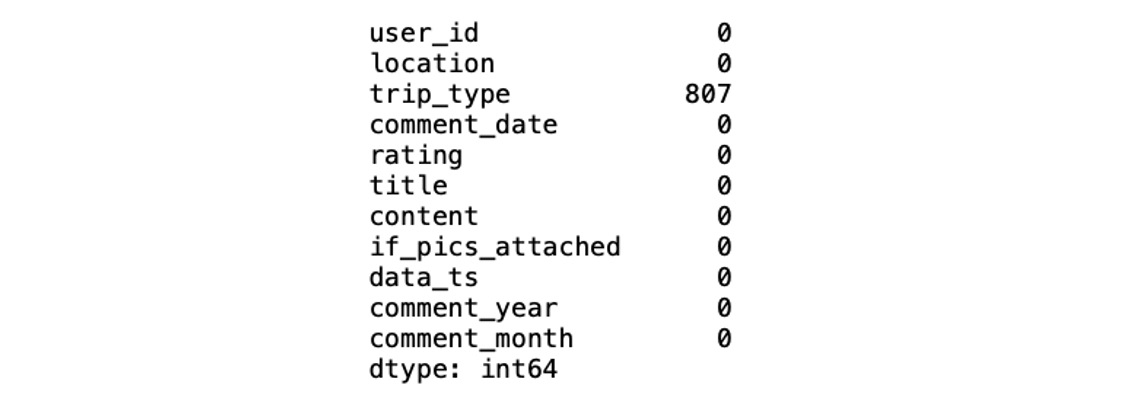### 3.处理旅行类型空值

```df.groupby(['trip_type']).size()
```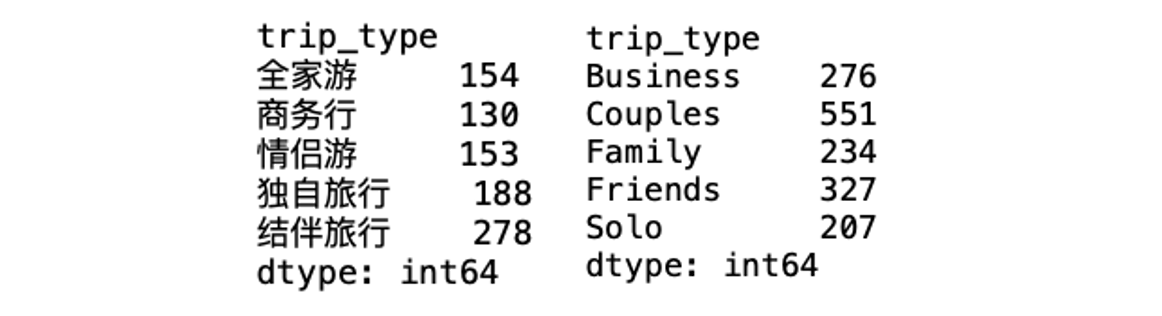```df['trip_type'] = df['trip_type'].fillna('未知')
```

```df['trip_type'] = df['trip_type'].fillna('NA')
```

```zh_trip_type = ['全家游', '商务行', '情侣游', '独自旅行', '结伴旅行', '未知']
en_trip_type = ['Family', 'Business', 'Couples', 'Solo', 'Friends', 'NA']
trip_type_df = pd.DataFrame({'zh_type':zh_trip_type, 'en_type':en_trip_type})
```

```trip_type_df.to_sql("tripadvisor_TripType_lookup", engine, if_exists="replace", index=False)
```

### 4.处理英文评论数据中用户所在地信息

• 如果地区信息用逗号隔开，前一个词为城市，后一个词为国家/省份
• 如果没有逗号，则默认该信息为国家信息

• 将缩写的国家/省份提取出来并单独处理（以美国为主，用户在填写地区信息时只填写州名）
• 查看除缩写以外的国家信息，如国家名称未出现在国家列表里，则认为是城市信息
• 国家字段中出现的城市名错填（如大型城市）和拼写错误问题，则手动修改处理

```country_file = open("countries.txt", "r")
country_list = country_data.split("\n")
countries_lower = [x.lower() for x in country_list]

```

```def formating_country_info(s_input):
if s_input is None: #若字符串输入为空值，返回空值
return None
if s_input.strip().lower() in countries_lower: #若字符串输入在国家列表中，返回国家名
c_index = countries_lower.index(s_input.strip().lower())
return country_list[c_index]
else:
if len(s_input) == 2: #若输入为缩写，在美国州名、墨西哥省名和英国缩写中查找，若可以找到，返回对应国家名称
if s_input.strip().upper() in state_code["code"].to_list():
return "United States"
elif s_input.strip().upper() == "UK":
return "United Kingdom"
elif s_input.strip().upper() in ("RJ", "GO", "CE"):
return "Mexico"
elif s_input.strip().upper() in ("SP", "SG"):
return "Singapore"
else:
# could not detect country info
return None
else: #其他情况，需要手动修改国家名称
if s_input.strip().lower() == "caior":
return "Egypt"
else:
return None
```

```df["location_country"] = df["location_country"].apply(formating_country_info)
```

```df["location_country"].isnull().sum()
```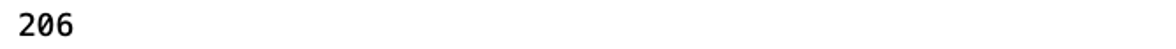```def check_if_country_info(city_list):
clean_list = []
country_fill_list = []
for city in city_list:
if city is None:
clean_list.append(None)
country_fill_list.append(None)
elif city.strip().lower() in countries_lower: #如城市变量中出现的是国家名，记录国家名称
c_index = countries_lower.index(city.strip().lower())
country_name = country_list[c_index]
if country_name == "Singapore": #如城市名为新加坡，保留城市名，如不是则将原先的城市名转换为空值
clean_list.append(country_name)
else:
clean_list.append(None)
country_fill_list.append(country_name)
else:
# format city string
city_name = city.strip().lower().capitalize()
clean_list.append(city_name)
country_fill_list.append(None)
return clean_list, country_fill_list
```

```city_list, country_fillin = check_if_country_info(df["location_city"].to_list())
```

```df["country_fill_temp"] = country_fillin
```

```df["location_city"] = city_list
df["location_country"] = df["location_country"].fillna(df["country_fill_temp"])
df = df.drop(columns=["country_fill_temp"])
```